•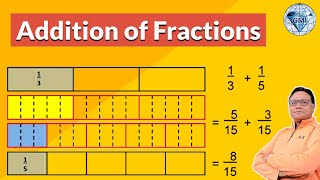WatchHow To Add Fractions with different Denominators
•WatchHow to Subtract Fractions with different Denominators
•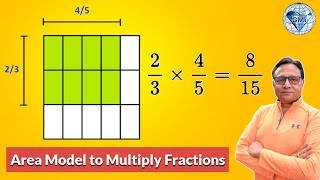WatchMultiply Fractions
•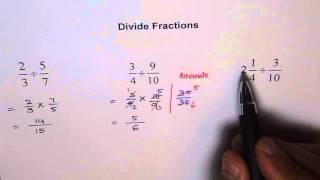WatchDivide Fractions by Fractions
•WatchWhat Are Mixed Fractions
•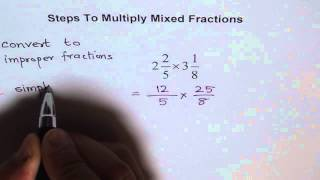WatchHow To Multiply Mixed Fractions
•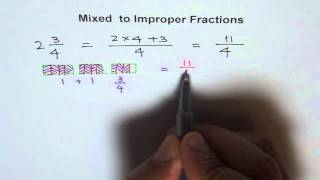WatchConvert Mixed Number To Improper Fraction
•WatchHow To Add Mixed Fractions
•WatchImproper Fraction and Mixed Fraction
•WatchConvert Improper To Mixed Fraction
•WatchAdd and Subtract Fractions
•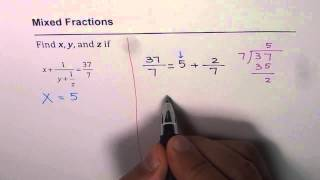Watch41 Mixed Fraction as Fraction of Fraction
•Watch42 Multi Layer Mixed Fraction as Fraction of Fraction
•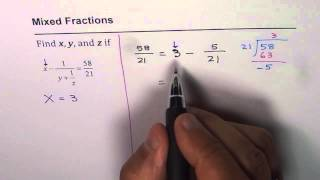Watch43 Mixed Fraction As Fraction of Fraction
•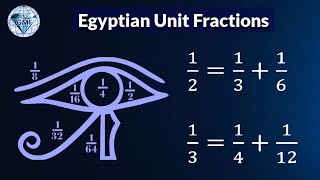WatchEgyptian Unit Fractions write 1/n as sum of two fractions with unit numerator
•WatchNumbers 1 to 9 Form Equivalent Fractions
•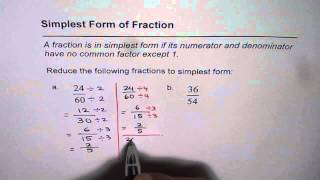Watch01 Reduce Fractions to Simplest Form Lowest Term
•Watch02 Reduce Fractions to Lowest Term or Simplest Form
•Watch03 Reduce Fraction to Simplest Form
•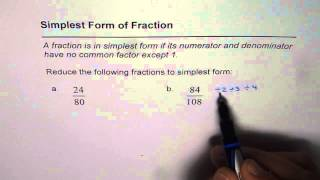Watch04 Simplest Form of Fraction
•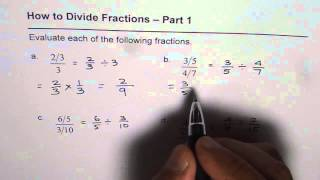WatchLearn How to Divide Fractions by Fractions
•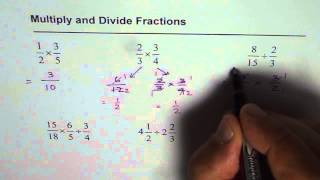Watch08 Multiply Divide Fractions
•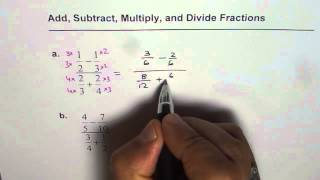WatchFraction Operations Add Subtract Multiply and Divide
•WatchPractice Order of Operation with Fractions
•WatchHow to Add and Divided Fractions
•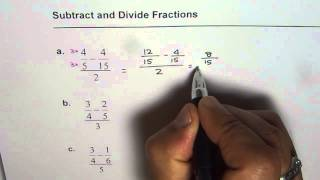WatchHow to Subtract and Divide Fractions
•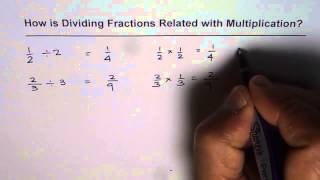WatchHow is Dividing Fractions Related with Multiplying